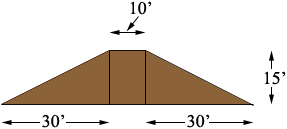SEARCH HOMEMath Central Quandaries & QueriesQuestion from Wes, a teacher: I need a levee with a 2:1 slope on both sides (front and back), the length of the levee is 290 feet, the height of the levee is 15 feet, and the width of the levee, at the top, is 10 feet. How do I figure out how many CUBIC YARDS of dirt I need to make the levee? And what formula do I use in future to figure this out easily?Wes,

I drew a cross-section of the levee.The volume of the levee is the area of the cross-section times the length of 290 feet. The cross-section can be seen as three pieces, a 10 foot by 15 foot rectangle and two right triangles, each with legs of length 15 feet and 30 feet. The area of a triangle is 1/2 × base × height so the area of the cross section is

(10 × 15) + (1/2 × 30 × 15) + (1/2 × 30 × 15) = 40 × 15 square feet.

Thus the volume of the levee is

290 × 40 × 15 cubic feet.

But you want the volume in cubic yards so I would convert each measurement to yards before performing the calculation. Thus the volume is

(290/3) × (40/3) × (15/3) cubic yards.

PennyMath Central is supported by the University of Regina and The Pacific Institute for the Mathematical Sciences.